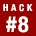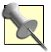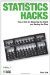# Hack 8. Power UpSuccess in social science research is typically defined by the discovery of a statistically significant finding. To increase the chances of finding something, anything, the primary goal of the statistically savvy super-scientist should be to increase power.

There are two potential pitfalls when conducting statistically based research. Scientists might decide that they have found something in a population when it really exists only in their sample. Conversely, scientists might find nothing in their sample when, in reality, there was a beautiful relationship in the population just waiting to be found.

The first problem is minimized by sampling in a way that represents the population [Hack #19]. The second problem is solved by increasing power.

#### Power

In social science research, a statistical analysis frequently determines whether a certain value observed in a sample is likely to have occurred by chance. This process is called a test of significance. Tests of significance produce a p-value (probability value), which is the probability that the sample value could have been drawn from a particular population of interest.

The lower the p-value, the more confident we are in our beliefs that we have achieved statistical significance and that our data reveals a relationship that exists not only in our sample but also in the whole population represented by that sample. Usually, a predetermined level of significance is chosen as a standard for what counts. If the eventual p-value is equal to or lower than that predetermined level of significance, then the researcher has achieved a level of significance.Statistical analyses and tests of significance are not limited to identifying relationships among variables, but the most common analyses (t tests, F tests, chi-squares, correlation coefficients, regression equations, etc.) usually serve this purpose. I talk about relationships here because they are the typical effect you're looking for.

The power of a statistical test is the probability that, given that there is a relationship among variables in the population, the statistical analysis will result in the decision that a level of significance has been achieved. Notice this is a conditional probability. There must be a relationship in the population to find; otherwise, power has no meaning.

Power is not the chance of finding a significant result; it is the chance of finding that relationship if it is there to find. The formula for power contains three components:

• Sample size

• The predetermined level of significance (p-value) to beat (be less than)

• The effect size (the size of the relationship in the population)

#### Conducting a Power Analysis

Let's say we want to compare two different sample groups and see whether they are different enough that there is likely a real difference in the populations they represent. For example, suppose you want to know whether men or women sleep more.

The design is fairly straightforward. Create two samples of people: one group of men and one group of women. Then, survey both groups and ask them the typical number of hours of sleep they get each night. To find any real differences, though, how many people do you need to survey? This is a power question.A t test compares the mean performance of two sample groups of scores to see whether there is a significant difference [Hack #17]. In this case, statistical significance means that the difference between scores in the two populations represented by the two sample groups is probably greater than zero.

Before a study begins, a researcher can determine the power of the statistical analysis that will be used. Two of the three pieces needed to calculate power are already known before the study begins: you can decide the sample size and choose the predetermined level of significance. What you can't know is the true size of the relationship between the variables, because data for the planned research has not yet been generated.

The size of the relationship among the variables of interest (i.e., the effect size) can be estimated by the researcher before the study begins; power also can be estimated before the study begins. Usually, the researcher decides on the smallest relationship size that would be considered important or interesting to find.

Once these three pieces (sample size, level of significance, and effect size) are determined, the fourth piece (power) can be calculated. In fact, setting the level of any three of these four pieces allows for calculation of the fourth piece. For example, a researcher often knows the power she would like an analysis to have, the effect size she wants to be declared statistically significant, and the preset level of significance she will choose. With this information, the researcher can calculate the necessary sample size.For estimating power, researchers often use a standard accepted procedure that identifies a power goal of .80 and assigns a preset level of significance of .05. A power of .80 means that a researcher will find a relationship or effect in her sample 80 percent of the time if there is such a relationship in the population from which the sample was drawn.

The effect size (or index of relationship size [Hack #10]) with t tests is often expressed as the difference between the two means divided by the standard deviation in each group. This produces effect sizes in which .2 is considered small, .5 is considered medium, and .8 is considered large. The power analysis question is: how big a sample in each of the two groups (how many people) do I need in order to find a significant difference in test scores?

The actual formula for computing power is complex, and I won't present it here. In real life, computer software or a series of dense tables in the back of statistics books are used to estimate power. I have done the calculations for a series of options, though, and present them in Table 1-7. Notice that the key variables are effect size and sample size. By convention, I have kept power at .80 and level of significance at .05.

##### Table Necessary sample sizes for various effect sizes
Effect sizeSample size
.101,600
.20400
.30175
.40100
.5065
1.020

Imagine that you think the actual difference in your gender-and-sleep study will be real, but small. A difference of about .2 standard deviations between groups in t test analyses is considered small, so you might expect a .2 effect size. To find that small of an effect size, you need 400 people in each group! As the effect size increases, the necessary sample size gets smaller. If the population effect size is 1.0 (a very large effect size and a big difference between the two groups), 20 people per group would suffice.

#### Making Inferences About Beautiful Relationships

Scientists often rely on the use of statistical inference to reject or accept their research hypotheses. They usually suggest a null hypothesis that says there is no relationship among variables or differences between groups. If their sample data suggests that there is, in fact, a relationship between their variables in the population, they will reject the null hypothesis [Hack #4] and accept the alternative, their research hypothesis, as the best guess about reality.

Of course, mistakes can be made in this process. Table 1-8 identifies the possible types of errors that can be made in this hypothesis-testing game. Rejecting the null hypothesis when you should not is called a Type I error by statistical philosophers. Failing to reject the null when you should is called a Type II error.

##### Table Errors in hypothesis testing
ActionNull hypothesis is trueNull hypothesis is false
Reject null hypothesis Type I errorSignificant finding
Fail to reject nullCorrect decision Type II error

What you want to do as a smart scientist is avoid the two types of errors and produce a significant finding. Reaching a correct decision to not reject the null when the null is true is okay too, but not nearly as fun as a significant finding. "Spend your life in the upper-right quadrant of the table," my Uncle Frank used to say, "and you will be happy and wealthy beyond your wildest dreams!"

To have a good chance of reaching a statistically significant finding, one condition beyond your control must be true. The null hypothesis must be false, or your chances of "finding" something are slim. And, if you do "find" something, it's not really there, and you will be making a big errora Type I error. There must actually be a relationship among your research variables in the population for you to find it in your sample data.

So, fate decides whether you wind up in the column on the right in Table 1-8. Power is the chance of moving to the top of that column once you get there. In other words, power is the chance of correctly rejecting the null hypothesis when the null hypothesis is false.

#### Why It Works

This relationship between effect size and sample size makes sense. Think of an animal hiding in a haystack. (The animal is the effect size; just work with me on this metaphor, please.) It takes fewer observations (handfuls of hay) to find a big ol' effect size (like an elephant, say) than it would to find a tiny animal (like a cute baby otter, for instance). The number of people represents the number of observations, and big effect sizes hiding in populations are easier to find than smaller effect sizes.

The general relationship between effect size and sample size in power works the other way, too. Guess at your effect size, and just increase your sample size until you have the power you need. Remember, Table 1-7 assumes you want to have 80 percent power. You can always work with fewer people; you'll just have less power.

#### Where It Doesn't Work

It is important to remember that power is not the chance of success. It is not even the chance that a level of significance will be reached. It is the chance that a level of significance will be reached if all the values estimated by the researcher turn out to be correct. The hardest component of the formula to guess or set is the effect size in the population. A researcher seldom knows how big the thing is that he is looking for. After all, if he did know the size of the relationship between his research variables, there wouldn't be much reason to conduct the study, would there?Statistics Hacks: Tips & Tools for Measuring the World and Beating the Odds
ISBN: 0596101643
EAN: 2147483647
Year: 2004
Pages: 114
Authors: Bruce Frey

Similar book on Amazon